# Finding an equation of best fit in Desmos

//Finding an equation of best fit in Desmos

## Finding an equation of best fit in Desmos

1. Go to Desmos.com and choose Start Graphing.
2. In the upper left, choose Add Item > table.
3. Type your data in the table.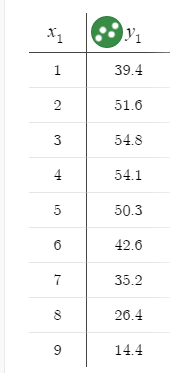4. Click on the wrench in the upper right to change the graph settings.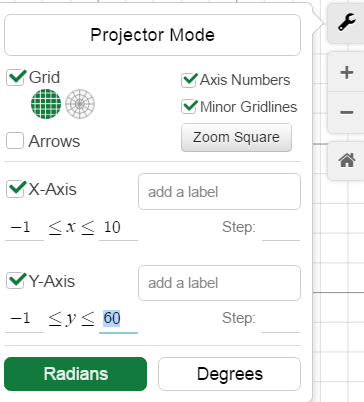6. Decide what type of equation the data represents. In the example above, the data appears to be quadratic.
7. In the input area, type y=a(x-h)^2 + k and press Enter. Sliders will be added for a, h, and k.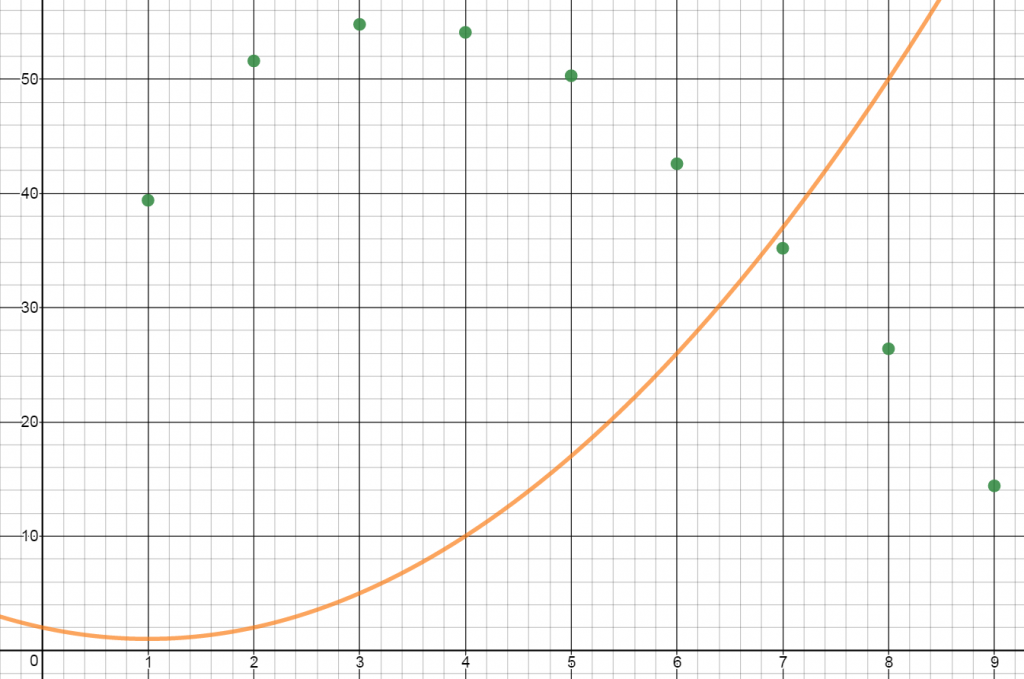8. Adjust the values of the sliders until the graph of the equation most closely fits your data points. You will likely need to change your slider settings. To do so, click on the gear icon to Edit List.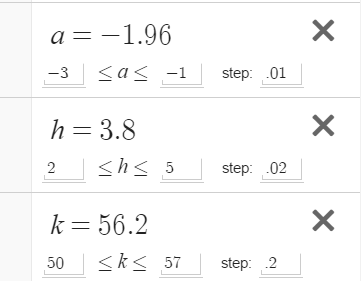9. The R-squared value is a statistical measure of how close the data are to a fitted regression line. The closer R2 is to 1, the better the curve matches the data. To have Desmos calculate your R2 value in a new input line type y1 ~ a(x1-h)^2+k. Desmos uses y1 to represent the y-value in a data table and x1 to represent the x-values in a table.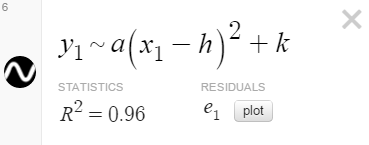10. Adjust your sliders until you get the highest possible value for R². Write down your equation of best fit.
11. To have Desmos create an equation of best fit, in the input bar, add a new equation y1~bx1^2+cx1+d. Compare the equation Desmos generated to yours.
By | 2017-11-13T22:01:55+00:00 September 24th, 2016|Uncategorized|16 Comments

### About the Author: Stacie Bender1.Mrs Lee Felix October 24, 2019 at 1:02 pm - Reply

•CL3 May 20, 2021 at 8:35 am - Reply

Take logs of both sides and fit as linear

•Alek May 30, 2021 at 6:49 am - Reply

y1~ab^x1

2.dali November 20, 2019 at 12:09 pm - Reply

or y1~mx1+b

•Genevieve Ingram June 10, 2020 at 1:12 pm - Reply

The equation that you listed will get you the LINE of best fit. This page helps you find the CURVE of best fit. Apologies for the misconception.

3.thanks April 13, 2021 at 12:07 am - Reply

omg you saved my life I looked for an hour

4.Grenon August 26, 2021 at 9:35 am - Reply

Thank you

5.Foxie October 18, 2021 at 2:08 pm - Reply

What about if it’s not a curve?

•owen March 22, 2022 at 5:35 pm - Reply

y1~mx1+b Is the line of best fit if that’s what you are looking for

6.Donshae March 8, 2022 at 1:36 pm - Reply

you put in y1~m 1/2 -bn1 –

7.Donshae March 8, 2022 at 1:37 pm - Reply

you put in y1~m 1/2

8.Daniella Stefan April 24, 2022 at 4:04 pm - Reply

Is it possible to do y=ax^2+bx+c?

•Josh October 18, 2022 at 12:56 pm - Reply

Yes, use y1 ~ ax1^2 + bx1 + c

•Alexi January 5, 2023 at 4:06 pm - Reply

Yes just put a subscript 1 on each x and y variable. And a squiggle line instead of equals.

9.Raven Velasquez November 8, 2022 at 12:33 pm - Reply

how do you do an eclipse (like an oval) of best fit?

10.